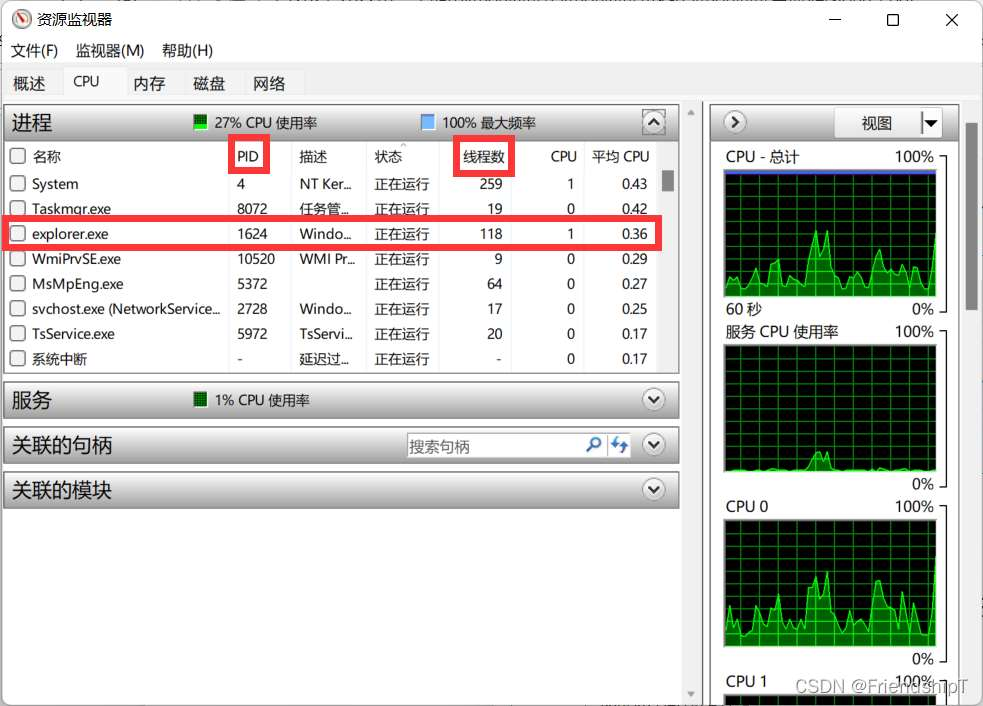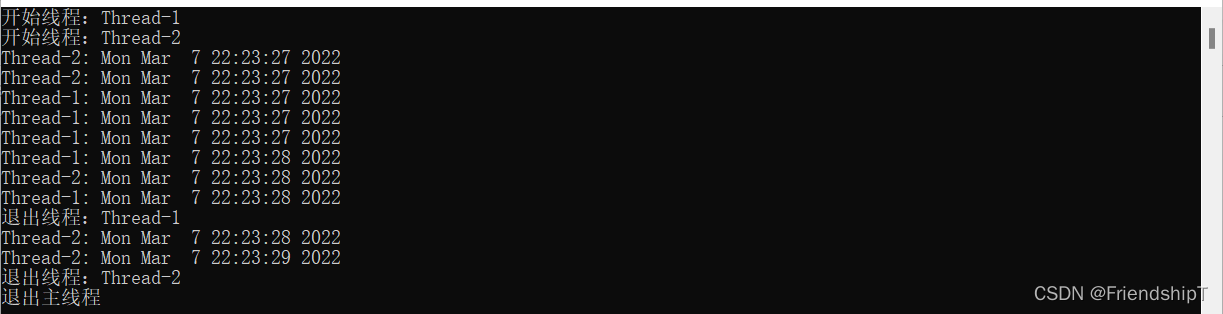# Python的進程,線程和協程實例詳解

更新時間：2022年03月10日 15:00:55   作者：FriendshipT

## 相關介紹

Python是一種跨平臺的計算機程序設計語言。是一個高層次的結合了解釋性、編譯性、互動性和面向對象的腳本語言。最初被設計用于編寫自動化腳本(shell)，隨著版本的不斷更新和語言新功能的添加，越多被用于獨立的、大型項目的開發。## 實驗環境

• Python 3.x （面向對象的高級語言）
• Multiprocessing（Python庫）
• Asyncio（Python庫）
• Time（Python庫）
• Random（Python庫）

## 進程1.首先要導入 multiprocessing 中的 Process；

2.創建一個 Process 對象；

3.創建 Process 對象時，可以傳遞參數；

4.使用 start()啟動進程；

5.結束進程。

```import os
from multiprocessing import Process
import time
def pro_func(name,age,**kwargs):
print("進程正在運行,name=%s, age=%d, pid=%d" %(name, age, os.getpid()))
print('kwargs參數值',kwargs)
time.sleep(0.1)
if __name__=="__main__":
p=Process(target=pro_func,args=('Friendship',18),kwargs={'愛好':'Python'})
print('啟動進程')
p.start()
print('程是否還在活著:',p.is_alive())# 判斷進程進程是否還在活著
time.sleep(0.5)
# 1 秒鐘之后，立刻結束進程
print('結束進程')
p.terminate() # 不管任務是否完成，立即終止進程
p.join() # 等待子進程執行結束
print('程是否還在活著:',p.is_alive())# 判斷進程進程是否還在活著
```### 多進程

```import os
import time
import random
from multiprocessing import Process,Queue
# 寫數據函數
def write(q):
for value in ['I','love','Python']:
print('在隊列里寫入 %s ' % value)
q.put(value)
time.sleep(random.random())
# 讀數據函數
while True:
if not q.empty():
value  = q.get(True)
print('從隊列中讀取 %s ' % value)
time.sleep(random.random())
else:
break
if __name__=="__main__": # 主進程
# 主進程創建 Queue，并傳給各個子進程
q=Queue()
# 創建兩個進程
pw=Process(target=write,args=(q,))
# 啟動子進程 pw
pw.start()
# 等待 pw結束
pw.join()
# 啟動子進程 pr
pr.start()
# 等待 pw結束
pr.join()
print('End!')
```### 用進程池對多進程進行操作

```from multiprocessing import Manager,Pool
import os,time,random
for i in range(q.qsize()):
print("read進程 從 Queue 獲取到消息：%s" % q.get(True))
def write(q):
print("write進程 啟動(%s),主進程為(%s)" % (os.getpid(), os.getppid()))
for i in "Python":
q.put(i)
if __name__=="__main__":
print("主進程(%s) start" % os.getpid())
q = Manager().Queue() # 使用 Manager 中的 Queue
# 定義一個進程池
po = Pool()
# Pool().apply_async(要調用的目標,(傳遞給目標的參數元祖,))
po.apply_async(write, (q,))
time.sleep(1) # 先讓上面的任務向 Queue 存入數據，然后再讓下面的任務開始從中取數據
po.close() # 關閉進程池，關閉后 po 不再接收新的請求
po.join() # 等待 po 中所有子進程執行完成，必須放在 close 語句之后
print("(%s) End!" % os.getpid())
```## 線程```import _thread
import time
import random
# 為線程定義一個函數
count = 0
while count < 5:
time.sleep(random.random())
count += 1
print ("%s: %s" % (threadName, time.ctime(time.time())))
# 創建兩個線程
try:
except:
print ("Error: 無法啟動線程")
while True:
pass
``````# 使用 threading 模塊創建線程
import time
import random
self.name = name
self.delay = random.random()
def run(self):
print ("開始線程：" + self.name)
print_time(self.name, 5)
print ("退出線程：" + self.name)
while count:
time.sleep(random.random())
print ("%s: %s" % (threadName, time.ctime(time.time())))
count -= 1
# 創建兩個線程
# 開啟新線程
print ("退出主線程")
```## 協程

• 協程：是一種用戶態的輕量級線程，協程的調度完全由用戶控制。協程擁有自己的寄存器上下文和棧。 協程調度切換時，將寄存器上下文和棧保存到其他地方，在切回來的時候，恢復先前保存的寄存器上下文和棧，直接操作棧則基本沒有內核切換的開銷，可以不加鎖的訪問全局變量，所以上下文的切換非?？?。
• 當出現IO阻塞的時候，由協程的調度器進行調度，通過將數據流立刻yield掉(主動讓出)，并且記錄當前棧上的數據，阻塞完后立刻再通過線程恢復棧，并把阻塞的結果放到這個線程上去跑，這樣看上去好像跟寫同步代碼沒有任何差別，這整個流程可以稱為coroutine。
• 由于協程的暫停完全由程序控制，發生在用戶態上;而線程的阻塞狀態是由操作系統內核來進行切換，發生在內核態上。因此，協程的開銷遠遠小于線程的開銷。

### 使用asyncio模塊實現

```import asyncio
import time
import random
async def work(msg):
print("收到的信息:'{}'".format(msg))
print("{}1{}".format("*"*10,"*"*10)) # 為了方便，展示結果
await asyncio.sleep(random.random())
print("{}2{}".format("*"*10,"*"*10)) # 為了方便，展示結果
print(msg)
async def main():
# 創建兩個任務對象(協程)，并加入到事件循環中
print("開始時間: {}".format(time.asctime(time.localtime(time.time()))))
await Coroutines1  # 此時并發運行Coroutines1和Coroutines2
print("{}3{}".format("*"*10,"*"*10)) # 為了方便，展示結果
await Coroutines2 # await相當于掛起當前任務，去執行其他任務，此時是堵塞的
print("{}4{}".format("*"*10,"*"*10)) # 為了方便，展示結果
print("結束時間:{}".format(time.asctime(time.localtime(time.time()))))
asyncio.run(main())# asyncio.run(main())創建一個事件循環，并以main為主要程序入口
```## 總結

• 進程：一個運行的程序（代碼）就是一個進程，沒有運行的代碼叫程序，進程是系統資源分配的最小單位，進程擁有自己獨立的內存空間，所以進程間數據不共享，開銷大。
• 線程： 調度執行的最小單位，也叫執行路徑，不能獨立存在，依賴進程存在一個進程至少有一個線程，叫主線程，而多個線程共享內存(數據共享，共享全局變量)，從而極大地提高了程序的運行效率。
• 協程：是一種用戶態的輕量級線程，協程的調度完全由用戶控制。協程擁有自己的寄存器上下文和棧。 協程調度切換時，將寄存器上下文和棧保存到其他地方，在切回來的時候，恢復先前保存的寄存器上下文和棧，直接操作棧則基本沒有內核切換的開銷，可以不加鎖的訪問全局變量，所以上下文的切換非?？?。